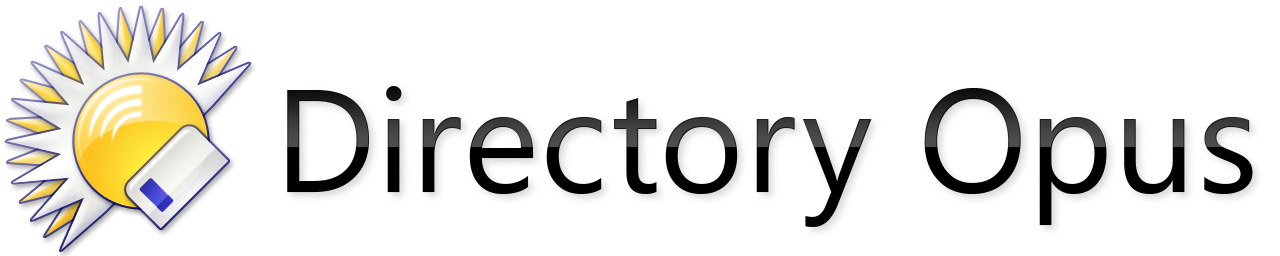# Dimensions for iconset

I began working on a full new icon set (default and additional icons). Very early concept:

The regular icons are sized 22 and 32 pixels and even with scaling they look okay. But which resolutions would you recommend now for small and large icons?

The internal icon sets use 22, 32, 48 and 64 for different DPIs:

``````<?xml version="1.0" encoding="UTF-8"?>
<iconset name="default">
<display_name>...</display_name>
<artist>...</artist>
<set filename="ICONS_22.PNG" size="small" width="22" height="22">
<dpi base="100">
<scale factor="100" filename="ICONS_22.PNG" width="22" height="22" no_scale_min="0" no_scale_max="125" />
<scale factor="150" filename="ICONS_32.PNG" width="32" height="32" no_scale_min="126" no_scale_max="175" />
<scale factor="200" filename="ICONS_48.PNG" width="48" height="48" />
<scale factor="300" filename="ICONS_64.PNG" width="64" height="64" />
</dpi>
<icon col="1" name="empty" row="1" />
...
</set>
<set filename="ICONS_32.PNG" size="large" width="32" height="32">
<dpi base="100">
<scale factor="100" filename="ICONS_32.PNG" width="32" height="32" no_scale_min="0" no_scale_max="125" />
<scale factor="150" filename="ICONS_48.PNG" width="48" height="48" no_scale_min="126" no_scale_max="175" />
<scale factor="200" filename="ICONS_64.PNG" width="64" height="64" />
</dpi>
<icon col="1" name="empty" row="1" />
...
</set>
</iconset>
``````
1 Like